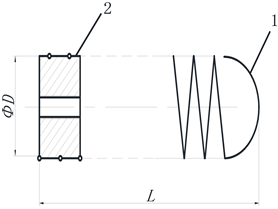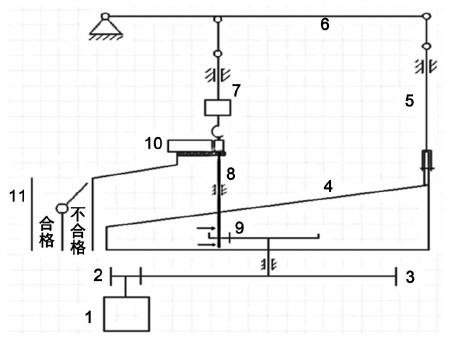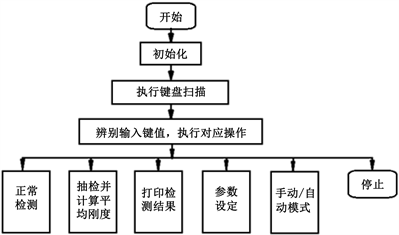﻿ 特型弹簧智能刚度检测仪的研制

# 特型弹簧智能刚度检测仪的研制Development of Intelligent Detector for Special Spring Stiffness

Abstract: An intelligent detector for special tension spring stiffness used in mechanical thermostat was de-veloped. Thought analyzing the structure of thermostat spring, the mechanical part of stiffness detector was designed which was used to clamp and pull the spring automatically. The detector used 8051 SCM as the controlling core, and a high-precision force sensor was used to collect force data. The stiffness value of springs was detected quickly and accurately, and the springs were classified according to test data. This detector improves the detection efficiency and accuracy ef-fectively. Detection rate can reach more than 400/hour. Measurement error is ±0.02 N/mm.

1. 引言1——挂钩，2——螺母。

Figure 1. Temperature controller spring

2. 检测装置工作原理与机构设计

2.1. 刚度检测原理分析

$F=k×\Delta l$ (1)

${F}_{1}={k}_{1}×\Delta {l}_{1}$ (2)

${F}_{2}={k}_{2}×\Delta {l}_{2}$ (3)

${F}_{2}-{F}_{1}={k}_{2}×\Delta {l}_{2}-{k}_{1}×\Delta {l}_{1}=k{\left(\Delta {l}_{2}-\Delta l\right)}_{1}$

$k=\left({F}_{2}-{F}_{1}\right)/\left(\Delta {l}_{2}-\Delta {l}_{1}\right)$ (4)

2.2. 检测装置机构设计1——步进电机，2——小齿轮，3——大齿轮，4——端面凸轮，5——推杆，6——杠杆， 7——挂钩，8——螺杆，9——摩擦轮，10——送簧机构，11——分拣装置。

Figure 2. Detection device mechanism diagramFigure 3. 3D model of the stiffness detector

3. 测控系统设计Figure 4. System service process

4. 结论

 胡琴. 电气自动检测技术的现状与发展[J]. 硅谷, 2013(11): 10.

 赵辉, 俞朴. 微小尺寸弹性零件刚度自动检测方法的研究[J]. 仪器仪表学报, 2001, 22(s1): 49-50.

 张宏建. 自动检测技术与装置[M]. 北京: 化学工业出版社, 2010.

 老盛林. 单片机应用技术[M]. 北京: 北京理工大学, 2018.

Top